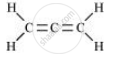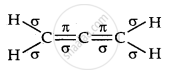CBSE (Science) Class 11CBSE
Share

# Indicate The σ And π Bonds in the Following Molecules: Ch2 = C = Ch2 - CBSE (Science) Class 11 - Chemistry

ConceptTetravalence of Carbon - Shapes of Organic Compounds

#### Question

Indicate the σ and π bonds in the following molecules:

CH= C = CH2

#### Solution 1

CH= C = CH2There are two C–C sigma (sigma_(C-C)) bonds, four C–H sigma (sigma_(C-H)) bonds, and two C=C pi (pi_(C-C)) bonds in the given compound

#### Solution 2Is there an error in this question or solution?

#### APPEARS IN

NCERT Solution for Chemistry Textbook for Class 11 (2018 to Current)
Chapter 12: Organic Chemistry - Some Basic Principles and Techniques
Q: 2.4 | Page no. 361
Solution Indicate The σ And π Bonds in the Following Molecules: Ch2 = C = Ch2 Concept: Tetravalence of Carbon - Shapes of Organic Compounds.
S